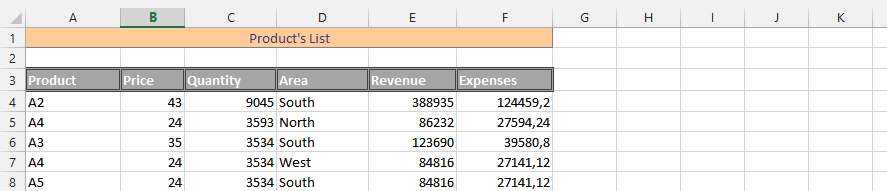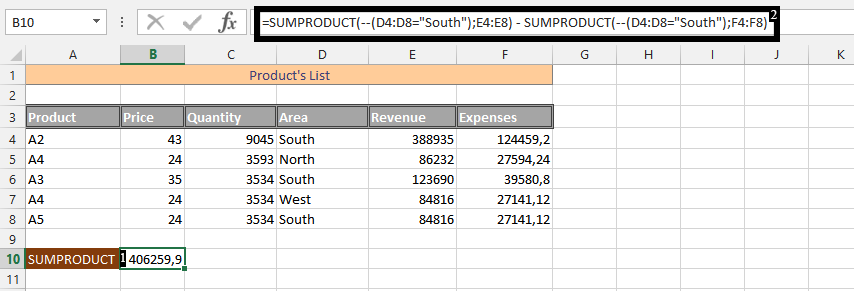#### Double dash in Sumproduct formula

In any given condition, where we are looking for something specific within our data. Like how much we have made from one particular product, or how much we have made from one of our stores. Or any condition that we just want an answer to some part of the whole data.

The double dash in the SUMPRODUCT formula is the perfect solution to get this result.

In this condition, we have sold our products in different places and have different products. But this time, we would like to know the net revenue from the South.

## Data preparation

Our data looks like this:## Inserting Sumproduct double dash formula

Click on an empty cell (1), and type in =SUMPRODUCT(–(D4:D8=”South”);E4:E8) – SUMPRODUCT(–(D4:D8=”South”);F4:F8) (2).Note: We’d subtract expenses from revenue for only the South. How you use the SUMPRODUCT with a double hyphen depends on what you would like the formula to obtain from the whole data set.

By using the double dash operator in a SUMPRODUCT formula, you can control which values are included in the calculation based on certain criteria, making the SUMPRODUCT function a powerful tool for data analysis and calculation in Excel.

Now you can use a double hyphen in Excel.